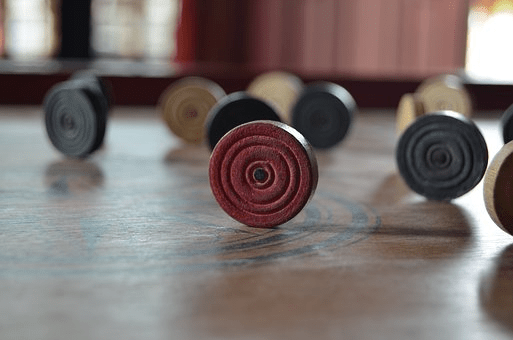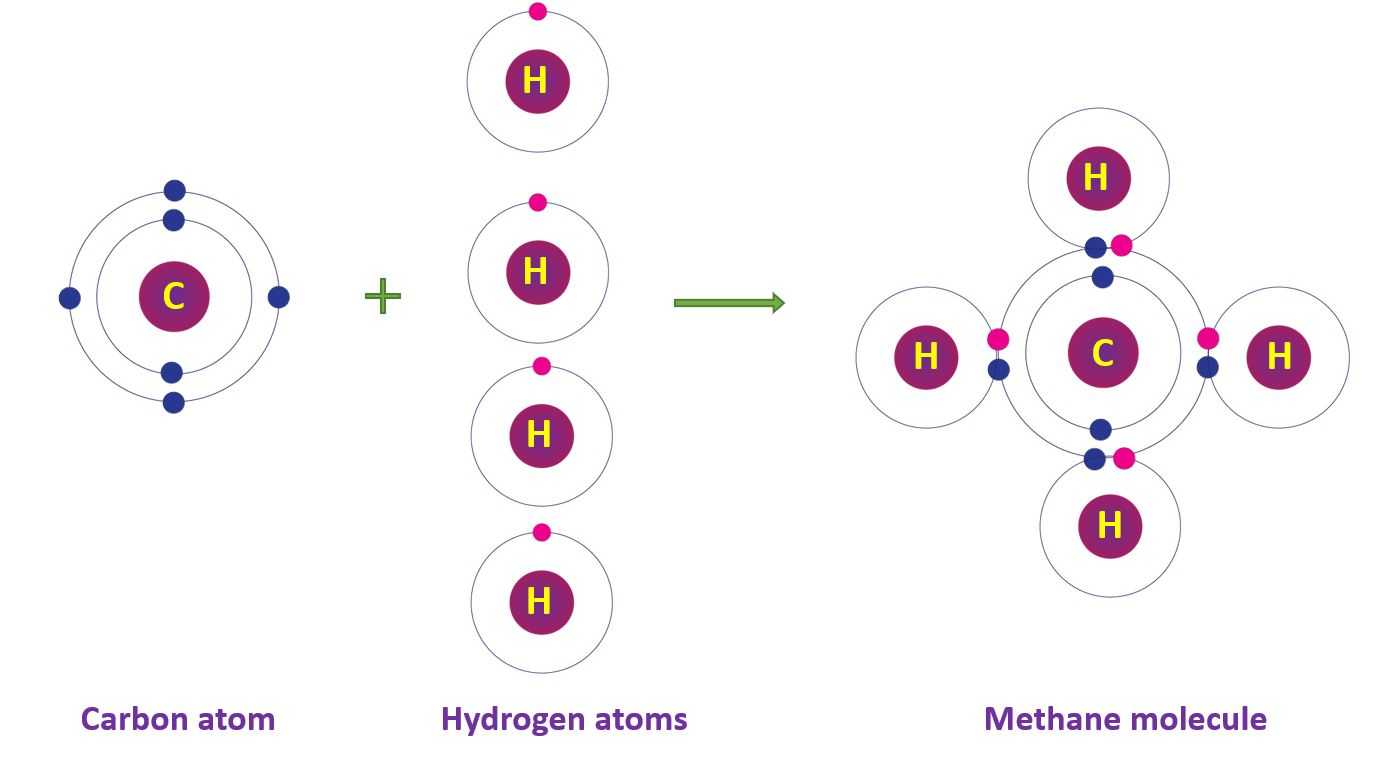# Newton’s laws of motion

What should we do to move a stationary body? We must exert force in the direction in which we want the body to move. Does it begin to move as soon as we apply force?

Sir Issac Newton, the famed physicist, explored in thoroughly what we experience with force and motion. Based on his research, he developed three laws of motion.

## Newton’s first law

Bodies at rest remain stationary until an unbalanced force is applied to them, while bodies in motion continue to move at uniform velocities.

Newton’s First Law of Motion, often known as the law of inertia, states that an object at rest will remain at rest, and an object in motion will continue to move at a constant velocity unless acted on by an external force.

In simpler terms, this means that an object will maintain its current state of motion unless an external force acts upon it. For example, if an object is at rest, it will remain at rest unless a force is applied to move it. Similarly, if an object is moving at a constant speed in a straight line, it will continue to do so unless acted upon by a force.

## Explain Newton’s first law with examples

Bodies at rest do not begin to move unless external forces act on them. We often notice that moving bodies come to rest without any external force being applied to them. Here’s an example to help clarify the point.

A ball rolling on a flat surface will continue to roll at a constant speed in a straight line unless it encounters friction or is acted upon by another force.

When a car suddenly stops, passengers inside the car will continue to move forward at the same speed until they are stopped by their seatbelts or the dashboard.

An ice skater gliding on the ice will continue to glide at a constant speed and in a straight line until they encounter friction or are acted upon by an external force.

Consider the instance of striking the carom disc with your fingernail on a carom board. The disc would then move a short distance before coming to rest. If we apply some talcum powder to the carom board to smooth it out, and then repeat the earlier motion by striking the disc with roughly the same force as before, the disc will go a significantly longer distance before coming to rest.The effect of the talcum powder on the board is to lessen the carom board’s resistive forces on the disc. Friction is the resistive force that opposes an object’s motion on a surface. If we could somehow reduce the frictional force to zero, the disc would move without stop.

Assume a person is standing on a moving bus with nothing to support them. If the bus comes to a rapid halt by using the brakes, the passengers will fall forward.

Because no such force is applied to his upper body, its velocity pushes him forward. He is thrown forward as a result of this.Assume the above passenger is sitting in a bus. If the bus begins to move without his awareness, the passenger will now fall backward. Once the bus begins to move, it exerts a force on the passenger’s feet, causing the lower part of his body to accelerate. The upper part of his body, on the other hand, lacks this velocity and remains at rest, causing him to fall back.

## Why should we wear a seat belt when traveling in a vehicle?Seat belts must be worn when riding in a vehicle to prevent passengers from falling forward when the brakes are applied. Because the seat belt exerts a force on the top part of his body as well, his entire body remains at the vehicle’s pace even when the brakes are applied. When no belts are worn, the upper body has some velocity and tends to go forward.

## Newton’s second law

According to Newton’s Second Law of Motion, an object’s acceleration is directly proportional to the force exerted on it and inversely proportional to its mass. This indicates that if an object is subjected to a net force, it will accelerate in the direction of the force, with the magnitude of the acceleration determined by the force’s strength and the mass of the object.

### The mathematical representation of Newton’s Second Law

The mathematical representation of Newton’s Second Law is expressed as:

F = ma

Where F is the net force exerted, m is the mass of the object, and an is its acceleration.

In simpler terms, this means that the force applied to an object will determine how much the object accelerates. If a greater force is applied, the object will experience a greater acceleration. Conversely, if the object has a greater mass, it will require a larger force to produce the same amount of acceleration.

When a force acts on an object, the force accelerates the object. If the force remains constant, the acceleration decreases with increasing mass. With a constant force, the acceleration increases as the mass lowers and decreases as the mass increases.

## Examples to illustrate Newton’s Second Law:

### Calculating acceleration by using Newton’s Second Law

If you push a shopping cart with a force of 10 Newtons, and the cart has a mass of 50 kg, the acceleration of the cart can be calculated using F = ma. In this case, the acceleration would be 0.2 meters per second squared.

If you throw a baseball with a force of 20 Newtons, and the baseball has a mass of 0.15 kg, the acceleration of the baseball can be calculated using F = ma. In this case, the acceleration would be 133 meters per second squared.

If you apply a force of 500 Newtons to a car with a mass of 1000 kg, the acceleration of the car can be calculated using F = ma. In this case, the acceleration would be 0.5 meters per second squared.

### Newton’s Second Law helps us to,

force applied to the object is related to its mass and acceleration. Newton’s Second Law helps us to understand how objects will move in response to applied forces and how to calculate their resulting motion.

## Newton’s third law

Newton’s Third Law of Motion states that for every action, there is an equal and opposite reaction. An action is defined as a force exerted by one object on another. The reaction is therefore a force applied by the second object on the first object. This means that when an object exerts a force on another object, the second object exerts an equal and opposite force back on the first object.

## Examples to illustrate Newton’s Third Law:

When a person jumps off a diving board, the person pushes down on the board with a force. According to Newton’s Third Law, the board will push back up on the person with an equal and opposite force. This is what propels the person into the air.When a rocket engine fires, it expels hot gases out of the back of the rocket. According to Newton’s Third Law, the gases pushing out of the rocket engine produce an equal and opposite force pushing the rocket forward.When you row a boat, your oar pushes against the water, and the water pushes back on the oar with an equal and opposite force. This is what propels the boat forward.